# Mathematical Model of Measuring Monitoring and Temperature Control of Growing Vegetables in Greenhouses

Mathematical Model of Measuring Monitoring and Temperature Control of Growing Vegetables in Greenhouses

Ivan S. LaktionovOleksandr V. Vovna Maryna M. Kabanets Maryna A. Derzhevetska Anatolii A. Zori

Department of Electronic Engineering, Faculty of Computer-Integrated Technologies, Automatization, Electrical Engineering and Radio Electronics, SHEE' Donetsk National Technical University' of the Ministry of Education and Science of Ukraine, Shybankova sq., 2, Pokrovsk UA85300, Ukraine

Language Training Department, Faculty of Computer-Integrated Technologies, Automatization, Electrical Engineering and Radio Electronics, SHEE' Donetsk National Technical University' of the Ministry of Education and Science of Ukraine, Shybankova sq., 2, Pokrovsk UA85300, Ukraine

Department of Medical Physics and Information Technology, Donetsk National Medical University, Railway Station str., 27, Lyman UA84401, Ukraine

Corresponding Author Email:
ivan.laktionov@donntu.edu.ua
Page:
325-336
|
DOI:
https://doi.org/10.18280/ijdne.150306
5 February 2020
|
Accepted:
17 February 2020
|
Published:
30 June 2020
| Citation

OPEN ACCESS

Abstract:

The main purpose of the article is to improve the mathematical model of the process of computerized measuring monitoring and adaptive fuzzy control of temperature modes of growing crops in greenhouse conditions. The results of the research were obtained using methods of physical and mathematical modelling, theory of differential equations of mathematical physics, theory of thermal conductivity, methods of structural-algorithmic synthesis of complex technical systems. The main purpose of the article was achieved by taking into account types and periods of vegetation of crops and factors of seasonality and engineering design of greenhouses, which allowed substantiating the functional diagram of the system of monitoring and control of temperature in the growing zone. The article establishes regularities of influence of natural sources and technical components of heat energy inputs and losses in greenhouses taking into account current requirements for technological modes of greenhouse operating, which allowed estimating the range of total specific heat energy sufficient for the production. Promising areas for further research of the developed model were proposed in order to increase the integral efficiency of greenhouse farms. The obtained research results can be used as a scientific and applied basis for substantiating ways to optimize energy consumption of industrial greenhouses.

Keywords:

energy balance, functional diagram, heat energy, greenhouse complex, microclimate, temperature

1. Introduction

1.1 Relevance of the research topic

One of the prerequisites for ensuring good nutrition of the population is uniform introduction of fresh vegetables and fruits into the diet throughout the year . Cost-effective and technically efficient functioning of industrial greenhouse complexes can contribute to the solution of the problem of seasonality of domestic vegetable production. In general, profitability of vegetable growing on protected grounds can be expressed in terms of energy intensity of production to the rate, quality, and volume of output greenhouse products. In order to ensure competitiveness of greenhouse enterprises in temperate and cold climates with a relatively high cost of energy, it is imperative to solve the problems of optimal and adaptive temperature control of cultivation using contemporary achievements in the field of digital, microprocessor, sensor, and information technology [2, 3]. Thus, conducting studies to justify the requirements for hardware and software for the subsystems of monitoring and control of greenhouse heating systems by mathematical modelling methods is an urgent scientific and applied task.

The scientific novelty of the obtained research results consists in the development of the nonlinear mathematical model of the process of measuring monitoring and adaptive control of temperature modes of cultivation by taking into account the types and periods of crops, as well as seasonality and climatic features of greenhouse locations. This fact allows to optimize plant growing modes in greenhouse conditions.

1.2 Analysis and generalization of known research results

At present, the problem of developing and substantiating mathematical models for monitoring and control of the temperature in the greenhouse growing area has been sufficiently addressed from theoretical and practical points of view. For example, the authors [4-8] propose to consider a greenhouse as a nonlinear system in which temperature is a dynamic characteristic and can be estimated on the basis of the energy balance equation. In the articles [9, 10] physical and mathematical foundations of forecasting the temperature dynamics in greenhouses are grounded on the basis of adaptive measuring monitoring of the greenhouse indoor microclimate and external climatic factors. In articles [11-13], the basic approaches to the implementation of simulation models of fuzzy control of temperature modes of cultivation on the basis of differential equations of heat and mass transfer are presented. In articles [14-16] basic scientific and theoretical provisions concerning physical, mathematical and simulation modelling of industrial greenhouse microclimate with the use of modern hardware and software solutions to the construction of computerized systems of monitoring and control of technological modes of crop cultivation on the protected grounds are presented. The results of the development of dynamic models of influence of microclimate parameters on the quality of growing vegetable crops under greenhouse conditions are presented in articles [17-19], as well as the main algorithms and results of numerical solution of the proposed models.

The fact of a significant number of the studies of the greenhouse microclimate monitoring and control systems using IoT and GWT technologies [20, 21] has been established.

These developments allow to optimize the structural and software organizations of the monitoring and control systems of the growing crops regimes. These results can be used as a basic during development of the mathematical model of measuring monitoring and temperature control of growing vegetables in greenhouses.

Analysis and logical generalization of the known research results in the investigated subject area revealed lack of scientists’ attention to the influence of types and periods of greenhouse crops vegetation, taking into account factors of climatic zones and seasonality of cultivation on technical and functional characteristics of monitoring and control of heating systems. This fact necessitates conducting research to justify the requirements for structural and algorithmic organization of such subsystems by mathematical modelling methods, which will increase economic and technical efficiency of the production process of growing vegetables in greenhouses.

1.3 Purpose, object and structure of the research

The main purpose of the article is to improve the mathematical model of the process of computerized measuring monitoring and adaptive temperature control of crop growing in greenhouse conditions by taking into account types and periods of crop vegetation and the seasonality factor, which will allow optimizing energy consumption of greenhouses.

The object of the study is regularities of the influence of informative parameters and destabilizing factors on the distribution of heat energy in the greenhouse growing zone.

Section 2 describes the basic materials and methods used in the development of the mathematical model; section 3 presents the basic quantitative and qualitative results of mathematical modelling of the process of temperature monitoring in greenhouses; section 4 outlines promising areas for further research; section 5 summarizes the main conclusions of the article.

2. Materials and Methods of the Research

2.1 General research methods

Theoretical and experimental studies on the development and justification of the mathematical model of the process of measuring monitoring and control of temperature modes of greenhouse crop cultivation were obtained using the provisions of physical and mathematical modelling, theory of differential equations of mathematical physics, theory of thermal conductivity, structural-synthetic algorithms. The main research results were obtained in the specialized laboratories “Information and Measurement Engineering and Metrology” and “Computer Technologies and Modelling” of the Department of Electronic Engineering of SHEE “Donetsk National Technical University” using certified and standardized equipment and software.

2.2 Basic research technique

Measuring temperature monitoring is a dynamic process, the characteristics of which are mainly determined by the exchange of energy between the indoor greenhouse growing area and the outside environment. In this case, the mathematical modelling of thermodynamic processes occurring in the greenhouse involves establishing and solving equations of mathematical physics.

Based on the analysis of the known research results on modelling temperature modes in greenhouses, it is established that the modern engineering design of industrial greenhouse complexes allows assuming during mathematical modelling of thermodynamic processes that the greenhouse is an object with a uniform temperature distribution in the cultivation zone. Therefore, the basic equation for mathematical modelling of measuring temperature monitoring is :

$\frac{\partial {{T}_{air\text{ }in}}\left( t \right)}{\partial t}=\frac{{{Q}_{total}}\left( t \right)}{{{V}_{in}}\cdot {{\rho }_{air}}\cdot {{C}_{\rho }}}$        (1)

where, $T_{\text {air in }}$ is temperature inside the greenhouse, $^{\circ} \mathrm{C} ; Q_{\text {total}}$ is total heat energy in the growing zone, $\mathrm{W} ; t$ is time, $\mathrm{h}$; $V_{\text {in }}$ is volume of the greenhouse growing zone, $\mathrm{m}^{3}$; ${{\rho }_{air}}$ is air density, $\mathrm{kg} \cdot \mathrm{m}^{-3} ; C_{\rho}$ is specific heat of air, J kg-1.-1.

Temperature dynamics in greenhouses is affected by different processes of heat and mass transfer. Therefore, in order to develop a relatively accurate and adequate model describing heat distribution in the greenhouse growing zone, it is important to investigate in detail the mechanisms of these processes. Thus, the basis of the developed mathematical model is the equation of the balance of mass and energy inside the greenhouse [11, 22]:

${{Q}_{total}}={{Q}_{gain}}-{{Q}_{loss}}$     (2)

where, Qgain is amount of energy supplied to the greenhouse, W; Qloss is amount of energy consumed in a greenhouse, W.

Tomatoes and cucumbers were selected as the basic types of greenhouse crops when designing the mathematical model. The study of the mathematical model was conducted for two typical growing seasons: before fruiting and during fruiting. The annual growing period was divided into two main cycles: autumn-winter and spring-summer.

2.3 Sources of heat energy

The amount of energy coming into the greenhouse is greatly influenced by the following physical processes and phenomena: energy from solar radiation; energy from the heating system; thermal component of energy from artificial systems of additional lighting [10, 11, 22]. Thus, in general, the amount of the energy supplied to the greenhouse can be calculated by the formula:

${{Q}_{gain}}={{Q}_{s}}+{{Q}_{h}}+{{Q}_{l}}$          (3)

where, Qs is useful solar energy supplied to the greenhouse, W; Qh is heat energy from the heating system, W; Ql is thermal component of energy from artificial systems of additional lighting, W.

One of the most important factors determining the input of heat energy to the greenhouse is solar radiation. The heat energy supplied to the greenhouse from the sun can be estimated by the following relation [23, 24]:

${{Q}_{s}}={{A}_{g}}\cdot \gamma \cdot \tau \cdot {{I}_{total}}$    (4)

where, Ag is the greenhouse surface area (roof and sidewalls), m2; γ is the constant of the fraction of solar radiation entering the greenhouse and causing the temperature increase, relative units; τ is the greenhouse surface capacity, relative units; Itotal is the solar energy falling on the greenhouse surface, W∙m-2.

At present, there are several theoretical and empirical models that describe the dynamics of solar radiation. On the basis of the analysis of the obtained simulation data, the necessity of refining the model of solar radiation distribution with consideration of seasonal and daily dynamics of the solar radiation  for temperate continental climate was established. For the spring-summer period, the average value of daylight hours is equal to 13 hours (from 6 a.m. to 7 p.m.) and 9 hours for autumn-winter period (from 8 a.m. to 4 p.m.) for GMT+3 time zone. On this basis, the analytic expression for describing the daily dynamics of the solar radiation, taking into account the seasonality, is as follows:

– For the spring-summer cultivation cycle:

\left\{ \begin{align} & {{I}_{total}}=\left| {{k}_{decr}}\left[ {{I}_{SC}}\cdot \left( \sin \left( \omega t-\varphi \right)+\sin \left( \omega \right) \right) \right] \right|,\text{ 6n}\le \text{t}\le \text{19n;} \\ & {{I}_{total}}=0,\text{ otherwise,} \\ \end{align} \right.         (5)

– for the autumn-winter cultivation cycle:

\left\{ \begin{align} & {{I}_{total}}=\left| {{k}_{decr}}\left[ {{I}_{SC}}\cdot \left( \sin \left( \omega t-\varphi \right)+\sin \left( \omega \right) \right) \right] \right|,\text{ 8n}\le \text{t}\le \text{16n;} \\ & {{I}_{total}}=0,\text{ otherwise,} \\ \end{align} \right.        (6)

where, kdecr is the coefficient of the solar radiation attenuation, which varies in the range from 0.47 to 0.85, relative units; Isc is the solar constant equal to 1367 W∙m-2; ω is cyclic frequency of change in the solar radiation, defined as 2π/24, rad.; φ is the argument of sinusoidal variation of solar radiation (for spring-summer period – 13 hours, for autumn-winter – 8 hours, (GMT+3)); n is the continuous number of the day in the calendar year.

When estimating the value of the useful solar energy supplied to the greenhouse, the numerical values of the parameters were assigned in accordance with Table 1. Typical sizes of greenhouses were selected based on current recommendations for modern engineering design.

The heat transfer rate from the stationary heating system to the air of the growing zone, depending on the structural characteristics can be calculated by the formula [10, 23]:

${{Q}_{h}}={{m}_{h.c.}}\cdot {{c}_{h.c.}}\cdot \left( {{T}_{direct}}-{{T}_{back}} \right)$       (7)

where, mh.c is heat carrier consumption, kg∙s-1; ch.c is specific heat capacity of the heat carrier, J∙kg-1∙℃-1; Tdirect is the heat carrier supply temperature, ℃; Tback is the temperature of the heat carrier removed from the heating system, ℃.

During the estimation of the value of heat energy coming into the greenhouse from the stationary heating systems, the numerical values of the parameters were assigned in accordance with Table 2.

The thermal energy component of artificial light systems can be estimated by taking into account the existing recommendations  by the formula:

${{Q}_{l}}={{E}_{l}}\cdot {{F}_{l}}\cdot {{q}_{l}}\cdot {{\eta }_{l}}$        (8)

where, El is the required level of illumination of the growing zone, lx; Fl is the area of the greenhouse under lying surface, which ranges from 0.2 to 0.3 for greenhouses of different design, m2; ql is the specific heat release indicator, W∙lx-1∙m-2; ηl is the share of heat entering the growing zone, relative units.

In estimating the value of the heat energy supplied to the greenhouse from stationary specialized Phyto-LED artificial illumination systems, the numerical values of the parameters were assigned in accordance with Table 3.

Table 1. Assigned values of useful solar energy modelling

 Parameter Accepted value Units Surface area (Ag) (typical values for temperate continental climate) 1500; 3000 m2 Solar radiation fraction constant (γ) 0.5 rel. units Greenhouse surface capacity (τ) 0.7 rel. units Solar radiation attenuation coefficient (kdecr) 0.65 rel. units

Table 2. Assigned values of modelling heat energy from greenhouse heating systems

 Parameter Accepted value Units Consumption of heat carrier (mh.c.) 10 kg∙s-1 Specific heat capacity of heat carrier (ch.c.) 4200 J∙kg-1∙℃-1 Permissible difference between temperatures of the forward and reverse branches of the heating system (Tdirect – Tback) 10 ℃

Table 3. Assigned values of modelling heat energy from artificial light systems

 Parameter Accepted value Units Minimum required level of illumination (El) 6 315 lx The area of the greenhouse underlying surface (Fl=Ag/4) 375; 750 m2 Specific heat release rate (ql) 0.018 W∙lx-1∙m-2 Share of heat entering the growing zone (ηl) 1 rel. units

2.4 Sources that cause heat loss

Losses of heat energy in the greenhouse are largely due to the following processes and phenomena: heat loss due to thermal conductivity; heat loss due to soil absorption capacity; heat transfer due to ventilation and infiltration; condensation heat transfer [10, 11, 22]. Therefore, in general terms, the amount of energy lost in the greenhouse can be calculated as follows:

${{Q}_{loss}}={{Q}_{k}}+{{Q}_{g}}+{{Q}_{v}}+{{Q}_{i}}+{{Q}_{c}}$        (9)

where, Qk is heat loss due to thermal conductivity, W; Qg is heat loss due to soil absorption capacity, W; Qv is heat transfer through ventilation, W; Qi is heat transfer through infiltration, W; Qc is condensation heat transfer, W.

Based on the analysis of the dependence (9), it can be seen that the loss of heat energy is caused by a considerable number of physical processes, one of which is the heat loss due to thermal conductivity. This component of the energy loss can be calculated using the following equation :

${{Q}_{k}}=h\cdot {{A}_{g}}\cdot \left( {{T}_{air\text{ }in}}-{{T}_{air\text{ }out}} \right)$        (10)

where, h is the heat transfer coefficient, W∙m-2∙℃-1; Ag is the greenhouse surface area, m2; Tair in is temperature in the greenhouse, ℃; Tair out is ambient temperature, ℃.

The coefficient of heat transfer, which is a part of the Eq. (10), can be calculated depending on the speed of movement of air flows in the greenhouse  by the formula:

$h=2,8+1,2\cdot {{v}_{air\text{ in}}}$      (11)

where, vair in is the velocity of air flow, m∙c-1.

The optimum temperature inside the greenhouse depends on the types and periods of vegetation of the crops grown. Statistics on the temperature dynamics over the last calendar year in Ukraine were obtained according to the official data of the Ukrainian Hydrometeorological Centre: for the autumn-winter cycle it is +3.77℃, for the spring-summer cycle it is +15.6℃.

Therefore, the numerical values of the parameters in accordance with Table 4 are assigned when estimating the values of heat energy losses caused by the heat transfer effect.

The next physical process to be taken into account when estimating heat losses in the greenhouse is the soil absorbing capacity. The estimated value of the heat loss due to the soil absorption capacity can be calculated by the formula [11, 22]:

${{Q}_{g}}=\frac{{{k}_{g}}}{{{Z}_{g}}}\cdot {{F}_{l}}\cdot \left( {{T}_{air\text{ }in}}-{{T}_{soil}} \right)$        (12)

where, kg is the coefficient of soil thermal conductivity, W∙ m-1∙℃-1; Zg is thickness of the soil layer, m; Fl is the area of the greenhouse underlying surface, m2; Tair in is greenhouse air temperature, ℃; Tsoil is soil temperature, ℃.

The calculation of the heat losses was carried out taking into account the current requirements for temperature modes of the gas-air environment and the greenhouse soil, the thickness of the soil layer and typical sizes of industrial greenhouse complexes, as shown in Table 5.

Table 4. Assigned values of modelling heat energy losses due to thermal conductivity

 Parameter Accepted value Units Velocity of the air flow (vair in) 1 m∙s-1 Surface area (Ag) 1500; 3000 m2 Average ambient temperature (Tair out) +3.77; +15.6 ºС

Table 5. Assigned values of modelling heat energy losses due to soil absorption capacity

 Parameter Accepted value Units Soil thermal conductivity coefficient (kg) 1.52 W∙m-1∙ºС-1 Area of the greenhouse underlying surface (Fl) 375; 750 m2 Thickness of the soil layer (Zg) 0.5 m Permissible temperature difference (Tair in–Tsoil) 3 ºС

The effects of heat loss from the crop growing zone under the influence of heat and wind pressure are ventilation (forced and natural) (Qv) and infiltration (Qi). Estimation of the heat loss through ventilation can be performed by the following formula [11, 27]:

${{Q}_{v}}=\frac{{{r}_{v}}\cdot {{V}_{g}}\cdot {{k}_{air}}\cdot \left( {{T}_{air\text{ }in}}-{{T}_{air\text{ }out}} \right)\cdot {{C}_{\rho }}\cdot {{\rho }_{air}}}{3600}$         (13)

where, rv is percentage of opening mechanisms of the ventilation system, relative units; Vg is greenhouse volume, m3; kair is multiplicity of air exchange, h-1; Сρ is specific heat of air, J∙kg-1∙℃-1; Tair in is greenhouse temperature, ℃; Tair out is ambient temperature, ℃; ρair is air density, kg∙m-3.

The numerical value of the heat loss indicator due to the infiltration process, which is caused by the greenhouse surface density, can be estimated by the following formula :

${{Q}_{i}}=\frac{0,5\cdot {{V}_{g}}\cdot {{k}_{air}}\cdot \left( {{T}_{air\text{ }in}}-{{T}_{air\text{ }out}} \right)}{3600}$           (14)

Thus, the total heat loss (Qv+i) caused by the processes of ventilation and infiltration can be found by the formula:

\begin{align} & {{Q}_{v+i}}=\frac{{{r}_{v}}\cdot {{V}_{g}}\cdot {{k}_{air}}\cdot \left( {{T}_{air\text{ }in}}-{{T}_{air\text{ }out}} \right)\cdot {{C}_{\rho }}\cdot {{\rho }_{air}}}{3600}+ \\ & +\frac{0,5\cdot {{V}_{g}}\cdot {{k}_{air}}\cdot \left( {{T}_{air\text{ }in}}-{{T}_{air\text{ }out}} \right)}{3600} \\ \end{align}      (15)

Numerous parameters were assigned during the simulation of the heat loss due to the processes of ventilation and infiltration of the greenhouse, including seasonal factors, climatic characteristics of the region, types and periods of crop vegetation, as well as the engineering design of greenhouses, as shown in Table 6.

The effect of condensation, which is caused by the constant release of moisture by plants, leads to the restriction of the supply of the heat energy to the greenhouse growing zone. The heat energy losses due to the effect of condensation on the greenhouse surface can be numerically estimated by taking into account the geometric characteristics of greenhouses and the seasonality factor according to the following formula :

${{Q}_{c}}={{C}_{}}\cdot {{L}_{v}}\cdot {{A}_{g}}$        (16)

where, Cc is rate of condensation on the greenhouse surface, which is due to the difference between external and internal temperature and humidity, kg∙s-1∙m-2; Lv is enthalpy of saturated vapor, J∙kg-1.

Table 6. Assigned values of modelling heat energy losses due to ventilation and infiltration

 Parameter Accepted value Units Specific heat capacity of air (Cρ) 1010 J∙kg-1∙℃-1 Average ambient temperature (Tair out) +3.77; +15.6 ℃ Air density (ρair) 1.292 kg∙m-3 Multiplicity of air exchange (kair) 2 h-1 Greenhouse volume (Vg=Ag∙hg/4) 1875; 3750 m3 Percentage of opening the mechanisms of the ventilation system (rv) 0.6 rel. units

Estimates of the rate of condensation on the greenhouse surface were obtained by the researchers  for a temperate continental climate, as shown in Table 7.

Table 7. Assigned values of modelling of heat energy losses due to condensation

 Parameter Accepted value Units Surface area (Ag) 1500; 3000 m2 Saturated vapor enthalpy (Lv) 2.45∙106 J∙kg-1 Condensation rate in winter (Cc) 2.5∙10-6 kg∙s-1∙m-2

The authors of the study  also found that the energy losses due to condensation increase if the greenhouse surface temperature is lower than the dew point temperature of the indoor air. Therefore, taking into account this effect, we can assume that the condensation rate in the spring-summer growing cycle is zero.

3. Research Results

3.1 Quantitative and qualitative modelling results

When estimating the value of the useful solar energy according to formulas (4)–(6), which enters the greenhouse, the results were obtained in the normalized to the maximum energy value (for greenhouses with the area of 1500 m2 – 378.5 kW, for greenhouses with the area of 3000 m2 – 757 kW), as shown in Figure 1. The obtained simulation results, which are shown in Figure 1, proved the need to take into account the seasonal and daily dynamics of the solar radiation when estimating the heat energy entering the greenhouse growing zone from the sun. The estimated value of the heat energy entering the greenhouse growing zone from stationary heat supply systems, which is calculated by formula (7), is 420 kW. The obtained results of calculations of heat energy component from the heat supply systems proved the necessity of their account during the research of the mathematical model of measurement monitoring of the growing zone temperature of industrial greenhouses.

## 1.pngFigure 1. Normalized characteristics of the distribution of the useful solar energy

When estimating the value of the heat energy entering the greenhouse from the stationary artificial lighting systems using formula (8), the following numerical results were established: 42.6 kW – for greenhouses with the area of 1,500 m2; 85.2 kW – for greenhouses with the area of 3,000 m2.

Therefore, the total amount of the heat energy supplied to the greenhouse, taking into account the above components, which are calculated by formulas (4) – (8), can be estimated by formula (3). The simulation of the process of heat energy supply to greenhouses also takes into account the requirements for duration of the photoperiod of artificial lighting of greenhouse crops, which must be at least 16 hours. Thus, at the qualitative level, the heat input to the greenhouse is as follows: the useful solar energy – see Figure 1; the heat energy from the heating system – 24 hours a day; the heat energy from artificial lighting systems – from 4 a.m. to 8 p.m. (GMT+3). The obtained simulation results are normalized to the maximum energy value (for greenhouses with the area of 1500 m2 is equal Qgain max=841.1 kW, for greenhouses with the area of 3000 m2Qgain max=1262.2 kW). The normalized graph of energy distribution taking into account the areas of greenhouses is shown in Figure 2.

Based on the analysis of the simulation results shown in Figure 2, the estimated ranges of the total heat energy changes for greenhouses with typical sizes of 1500 and 3000 m2, respectively, are: from 420 to 841.1 kW and from 420 kW to 1262.2 kW. The average rate of the heat energy increase / decrease was also established: for greenhouses with the area of 1500 m2 it is 46.8 kW∙h-1; for greenhouses with the area of 3000 m2 – 93.6 kW∙h-1.

The obtained graph of distribution of the estimated values of the heat energy losses on the basis of formulas (10) and (11) due to the heat transfer effect taking into account seasonality, geometric sizes of greenhouses and types and periods of vegetation of crops is shown in Figure 3.

The results of the calculations shown in Figure 3 prove the need to take into account the effect of the heat energy transfer from the growing zone to the environment during the mathematical modelling of the process of temperature monitoring.

The obtained results of the calculations of the heat energy losses due to the soil absorption capacity, which are estimated by formula (12), are as follows: for greenhouses with the area of 1500 m2 it is 3.42 kW, for greenhouses with the area of 3000 m2 – 6.84 kW, which is from 5 to 20% of energy losses due to the thermal conductivity of the greenhouse surface and requires further consideration during the mathematical modelling of the temperature monitoring process.

The obtained graphical view of the distribution of estimated values of the total heat losses due to ventilation effects (natural and mechanical) and infiltration, taking into account seasonality, geometric sizes of greenhouses and types and periods of vegetation on the basis of formula (15) is shown in Figure 4.

The results of the numerical simulations shown in Fig. 4, prove the need to take into account the effect of heat losses due to the ventilation and infiltration during the mathematical modelling of the process of temperature monitoring.

The results of calculations of the heat energy losses due to the effect of condensation based on formula (16) are as follows: for spring-summer cycle Qc =0 W, for autumn-winter – 12.7 kW (for greenhouses with the area of 1500 m2) and 25.7 kW (for greenhouses with the area of 3000 m2). The results of the calculations prove the need to take into account the effect of condensation during the mathematical modelling of the process of temperature monitoring of the greenhouse growing zone.

## 2.png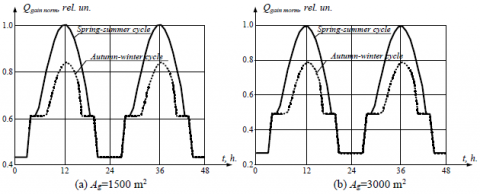Figure 2. Normalized characteristics of the distribution of the heat energy entering the greenhouse

## 3a.png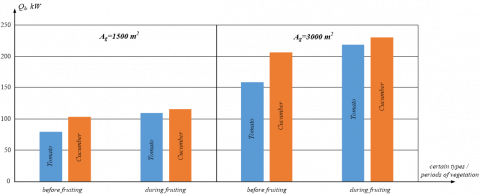(a) Autumn-winter cycle

## 3b.png(b) Spring-summer cycle

Figure 3. Heat energy losses due to the heat transfer effect

## 4a.png## 4b.pngFigure 4. Heat energy losses due to the effects of ventilation and infiltration

## 5.png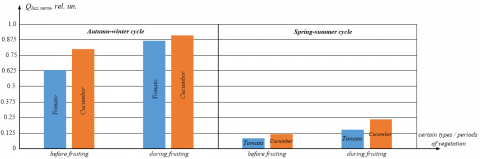Figure 5. Total heat energy losses

Therefore, the total amount of the heat energy lost in the greenhouse due to the effects of soil thermal conductivity, soil absorption capacity, ventilation, infiltration and condensation can be estimated by formula (9) based on the results of calculations by formulas (10) – (16). While modelling the process of heat energy losses from the greenhouse, the regulated requirements for the engineering design of greenhouses, the temperature-humidity modes of growing greenhouse crops and the seasonality factor were taken into account. The obtained results of modelling the distribution of total heat losses in the normalized graphical form (for greenhouses with the area of 1500 m2 it is equal to Qloss max=160 kW, for greenhouses with the area of 3000 m2Qloss max=320 kW) are shown in Figure 5.

Based on the analysis of the modelling results shown in Figure 5, the estimated ranges of changes in total heat loss for greenhouses with typical sizes of 1500 and 3000 m2, respectively, are: from 13.1 to 145.8 kW and from 26.2 to 291.6 kW, taking into account the seasonality factors and types and vegetation periods of growing crops.

Thus, taking into account the obtained values of the components of the input and losses of the heat energy in the greenhouse, the total heat energy (2) in the growing zone can be calculated. The estimated range of changes in the heat energy in the growing zone is obtained taking into account the following factors: seasonality, daily dynamics of solar radiation intensity, current requirements for temperature and humidity of certain types and periods of vegetation, engineering design of industrial greenhouses. The obtained results of mathematical modelling of heat energy dynamics in the graphical form are shown in Figures 6 and 7.

Based on the obtained data, which are shown in Figures 6 and 7, the dynamics of temperature in greenhouses by formula (1) can be estimated.

The differential equation is solved analytically under the following initial conditions: Tair in (0) = +3.77℃ – for the autumn-winter cycle and Tair in (0) = +15.6℃ – for the spring-summer cycle. The obtained results of modelling the temperature dynamics in the growing zone for cucumbers and tomatoes, taking into account the vegetation periods and seasonality are shown in Figures 8 and 9.

## 6a.png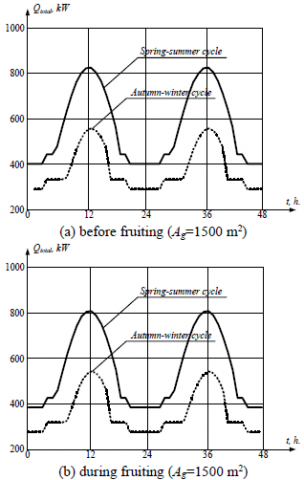## 6b.png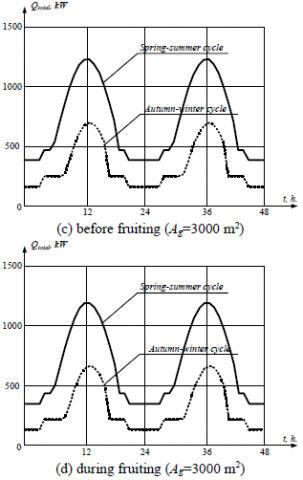Figure 6. Dependences of heat energy distribution in the greenhouse during cucumber growing

## 7a.png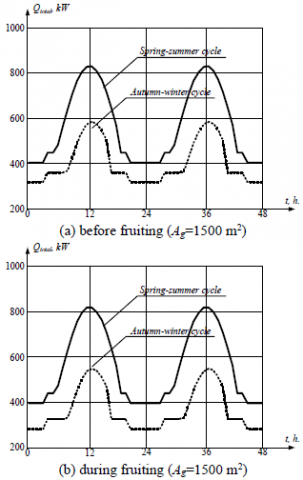## 7b.png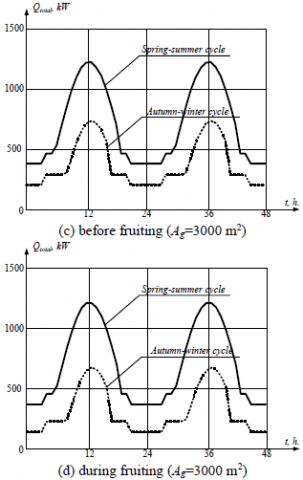Figure 7. Dependences of heat energy distribution in the greenhouse during tomato growing

By analysing the results of modelling the temperature dynamics in the greenhouse growing zone, which are shown in Figures 8 and 9, the following is established:

– During the cultivation of crops in the autumn-winter cycle under the condition of the heat energy input from the sun, the heating systems and the artificial lighting systems in the amount obtained under the above climatic conditions and source parameters is insufficient, which necessitates increasing the capacity of the heating systems and prompt control of them according to the results of measuring monitoring of internal and external influencing physical and chemical parameters;

– During the cultivation of crops in the spring-summer cycle under the condition of the heat energy input from the sun, the heating systems and the artificial lighting systems, as well as total energy losses in the amount obtained by the above of parameters is overestimated, which necessitates prompt control of ventilation and shading according to the results of measuring monitoring of internal and external influential physical and chemical parameters;

– The estimated values of the constant of the total specific heat energy, which is sufficient to ensure the regulated modes of cultivation of typical greenhouse crops , are as follows: for cucumbers before fruiting – 24.7 kW∙m-2, during fruiting – 25.9 kW∙m-2; for tomatoes before fruiting – 29.6 kW∙m-2, during fruiting – 22.2 kW∙m-2;

– Taking into account the types and periods of vegetation, seasonality and geometric dimensions of greenhouses leads to a complex function of the temperature dynamics, which makes it virtually impossible to implement control systems for growing temperatures using the classical laws of automatic control, and therefore there is a need to build subsystems for monitoring and control of air temperature in the greenhouse based on Fuzzy-logic.

3.2 Improved functional diagram

On the basis of the conducted research on the development of the mathematical model of measuring monitoring of air temperature in the greenhouse growing zone, the functional diagram of the measuring monitoring procedure for temperature modes of cultivation was refined, as specified in Figure 10.

Thus, the proposed functional diagram, which is shown in Figure 10, allows implementing the function of control of technological modes of cultivation, which is adaptive to types and periods of vegetation, taking into account seasonal factors and engineering design of greenhouses based on non-destructive measurement monitoring of basic characteristics of internal microclimate of greenhouses and external atmospheric parameters on a real-time basis.

## 8.pngFigure 8. Temperature dynamics during cucumber growing in industrial greenhouses

## 9.pngFigure 9. Temperature dynamics during tomato growing in industrial greenhouses

## 10.pngFigure 10. Refined functional diagram of measuring monitoring of the temperature modes of cultivation

4. Discussion and Suggestions for Future Investigations

The scientific novelty of the obtained research results consists in the development of the mathematical model of the process of measuring monitoring and adaptive control of temperature modes of cultivation by taking into account the types and periods of crops, as well as seasonality and climatic features of greenhouse locations.

The practical significance of the obtained research results lies in the substantiation of the functional diagram of the process of measuring monitoring and adaptive control of the main technological processes of growing greenhouse crops.

Promising areas for further research of the developed mathematical model are: development and testing in laboratory and field conditions of hardware and software of the measuring system, which implements the proposed mathematical model; developing the control unit of technological modes of cultivation and integrating it into the system on the basis of Fuzzy-logic; comprehensive assessment of technical and economic efficiency of the implementation of research results to the production conditions of industrial greenhouse complexes.

5. Conclusions

The article solves the topical scientific and applied problem of improving the mathematical model of computerized measuring monitoring and control of temperature modes of cultivation in greenhouse conditions taking into account the types and periods of crop vegetation and the seasonality factor, which allowed substantiating scientific and practical provisions of greenhouse energy consumption. The main quantitative and qualitative results of the research are:

– The regularities of the influence of energy from solar radiation, energy coming from the heating system and the thermal component of energy from artificial lighting systems on the total amount of heat energy generated in the greenhouse were established;

– The possible ranges of change of the total heat energy for greenhouses with typical sizes of 1500 and 3000 m2, respectively, were estimated: from 420 to 841.1 kW and from 420 kW to 1262.2 kW. The average rate of increase / decrease of the heat energy was also established: for the greenhouses with the area of 1500 m2 it is 46.8 kW∙h-1; for the greenhouses with the area of 3000 m2 it is 93.6 kW∙h-1.

– The regularities of the influence of heat losses due to the soil thermal conductivity, due to the soil absorptive capacity, heat transfer due to ventilation and infiltration and heat transfer by condensation on the total amount of the heat energy removed from the growing zone of greenhouses;

– The possible range of change in the loss of total heat energy for greenhouses with typical sizes of 1500 and 3000 m2, respectively, is: from 13.1 to 145.8 kW and from 26.2 kW to 291.6 kW;

– The estimated ranges of constant total specific heat energy are obtained, which is sufficient to ensure the regulated modes of cultivation of typical greenhouse crops: for cucumbers before fruiting – 24.7 kW∙m-2, during fruiting – 25.9 kW∙m-2; for tomatoes before fruiting – 29.6 kW∙m-2, during fruiting – 22.2 kW∙m-2;

– The functional diagram of the measurement monitoring process was developed, which allows implementing the function of adaptive control of the technological modes of cultivation depending on the types and periods of crop vegetation, taking into account the seasonality factors and engineering design of greenhouses on a real-time basis;

– The promising areas of research on hardware and software of non-destructive computerized monitoring and control of technological modes of cultivation using methods of physical and mathematical and simulation modelling are substantiated.

References

 FAO. http://www.fao.org/nutrition/education/food-dietary-guidelines/background/sustainable-dietary-guidelines/en/, accessed on April 28, 2020.

 Laktionov, I., Vovna, O., Zori, A. (2017). Concept of low cost computerized measuring system for microclimate parameters of greenhouses. Bulg. Journal of Agricultural Science, 23(4): 668-673.

 Laktionov, I.S., Vovna, O.V., Zori, A.A. (2017). Planning of remote experimental research on effects of greenhouse microclimate parameters on vegetable crop-producing. International Journal on Smart Sensing and Intelligent Systems, 10(4): 845-862. https://doi.org/10.21307/ijssis-2018-021

 Medina-Ruíz, C.A., Mercado-Luna, I.A., Soto-Zarazúa, G.M., Torres-Pacheco, I., Rico-García, E. (2011). Mathematical modeling on tomato plants: A review. African Journal of Agricultural Research, 6(33): 6745-6749. https://doi.org/10.5897/AJARX11.001

 Hemming, S., de Zwart, F., Elings, A., Righini, I., Petropoulou, A. (2019). Remote control of greenhouse vegetable production with artificial intelligence-greenhouse climate, irrigation, and crop production. Sensors, 19(8): 1-22. https://doi.org/10.3390/s19081807

 Caicedo-Ortiz, J.G., De-la-Hoz-Franco, E., Ortega, R.M., Pineres-Espitia, G., Combita-Nino, H., Estevez, F., Cama-Pinto, A. (2018). Monitoring system for agronomic variables based in WSN technology on cassava crops. Computers and Electronics in Agriculture, 145: 275-281. https://doi.org/10.1016/j.compag.2018.01.004

 Sanchez-Molina, J.A., Ming, L., Rodriguez, F., Guzman, J.L., Hui, W., Ya, X. (2017). Development and test verification of air temperature model for Chinese solar and Spainish Almeria-type greenhouses. Int J Agric & Biol. Eng., 10(4): 66-76. https://doi.org/10.25165/j.ijabe.20171004

 Ben Ali, R., Aridhi, E., Mami, A. (2015). Dynamic model of an agricultural greenhouse using Matlab-Simulink environment. In: 16th International Conference on Sciences and Techniques of Automatic Control and Computer Engineering (STA), Monastir, pp. 346-350. https://doi.org/10.1109/STA.2015.7505185

 Riahi, J., Vergura, S., Mezghani, D., Mami, A. (2020). Intelligent control of the microclimate of an agricultural greenhouse powered by a supporting PV system. Appl. Sci., 10(4): 1350. https://doi.org/10.3390/app10041350

 Shen, Y., Wei, R., Xu, L. (2018). Energy consumption prediction of a greenhouse and optimization of daily average temperature. Energies, 11(65): 1-17. https://doi.org/10.3390/en11010065

 Atia, D.M., El-madany, H.T. (2017). Analysis and design of greenhouse temperature control using adaptive neuro-fuzzy inference system. Journal of Electrical Systems and Information Technology, 4(1): 34-48. https://doi.org/10.1016/j.jesit.2016.10.014

 Faouzi, D., Bibi-Triki, N., Benmoussa, N., Bouhdjar, A., Abène, A. (2017). Optimizing the management and use of energy in the greenhouse by modeling the associated heating and cooling systems and implemented by an intelligent controller. International Journal of Computer Science and Technology, 8(2): 121-129.

 Mostakim, N., Mahmud, S., Jewel, K.H. (2020). A simulation based study of a greenhouse system with intelligent fuzzy logic. International Journal of Fuzzy Logic Systems, 10(1): 19-37. https://doi.org/10.5121/ijfls.2020.10102

 Laktionov, I., Vovna, O., Cherevko, О., Kozlovskaya, T. (2018). Mathematical model for monitoring carbon dioxide concentration in industrial greenhouses. Agronomy Research, 16(1): 134-146. https://doi.org/10.15159/AR.17.074

 Laktionov, I.S., Vovna, O.V., Zori, A.A., Lebediev, V.A. (2018). Results of simulation and physical modeling of the computerized monitoring and control system for greenhouse microclimate parameters. International Journal on Smart Sensing and Intelligent Systems, 11(1): 1-15. https://doi.org/10.21307/ijssis-2018-017

 Vovna, O.V., Laktionov, I.S., Koyfman, O.O., Stashkevych, I.I., Lebediev, V.A. (2020). Study of metrological characteristics of low-cost digital temperature sensors for greenhouse conditions. Serbian Journal of Electrical Engineering, 17(1): 1-20. https://doi.org/10.2298/SJEE2001001V

 Ehret, D.L., Hill, B.D., Helmer, T., Edwards, D.R. (2011). Neural network modeling of greenhouse tomato yield, growth and water use from automated crop monitoring data. Computers and Electronics in Agriculture, 79(1): 82-89. https://doi.org/10.1016/j.compag.2011.07.013

 Boote, K.J., Rybak, M.R., Scholberg, J.M., Jones, J.W. (2012). Improving the CROPGRO-Tomato model for predicting growth and yield response to temperature. HortScience, 47(8): 1038–1049. https://doi.org/10.21273/HORTSCI.47.8.1038

 Jones, J.W., Dayan, E., Allen, L.H., van Keulen, H., Challa, H. (1991). A dynamic tomato growth and yield model (TOMGRO). Transactions of the American Society of Agricultural and Biological Engineers, 34(2): 663-671.  https://doi.org/10.13031/2013.31715

 Wang, J., Zhou, J., Gu, R., Chen, M., Li, P. (2018). Manage system for internet of things of greenhouse based on GWT. Information Processing in Agriculture, 5(2): 269-278. https://doi.org/10.1016/j.inpa.2018.01.002

 Kitpo, N., Kugai, Y., Inoue, M., Yokemura, T., Satomura, S. (2019). Internet of things for greenhouse monitoring system using deep learning and bot notification services. In 2019 IEEE International Conference on Consumer Electronics (ICCE), Las Vegas, NV, USA, pp. 1-4. https://doi.org/10.1109/ICCE.2019.8661999

 Druma, A.M. (1998). Dynamic Climate Model of a Greenhouse. Report Geothermal Training Programme No. 3. The United Nations University, Reykjavik, Iceland.

 Emeish, M. (1999). Geothermal Heating System for Jordanian Greenhouses. Report 2 of Geothermal Training Programme. The United Nations University, Reykjavik, Iceland.

 Hanan, J.J. (1998). Greenhouses: Advanced Technology for Protected Horticulture. Boca Raton: CRC Press.

 Popovski, K., Popovska-Vasilevska, S. (1993). Heating greenhouses with geothermal energy. In: International Summer School, Skopje, pp. 1-17.

 Air Conditioning and Ventilation. https://www.hvac-school.ru/biblioteka/tepl_balans/metodika_rascheta/teploti_pomeshhen/, accessed on April 24, 2020.

 Kittas, C., Boulard, T., Papadakis, G. (1997). Natural ventilation of a greenhouse with ridge and side openings: sensitivity to temperature and wind effects. Am. Soc. Agric. Biol. Eng., 40: 415-425. https://doi.org/10.13031/2013.21268.

 Baudoin, W., Nersisyan, A., Shamilov, A., Hodder, A., Gutierrez, D., de Pascale, S., Nicola, S., Gruda, N., Urban, L., Tanny, J. (2017). Good Agricultural Practices for greenhouse vegetable production in the South East European countries. Rome: FAO.